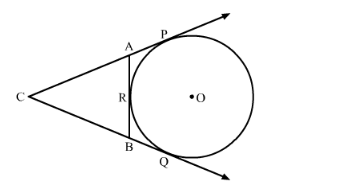# In the given figure, CP and CQ are tangents to a circle with centre O.

Question:

In the given figure, CP and CQ are tangents to a circle with centre OARB is another tangent touching the circle at R. If CP = 11 cm and BC = 7 cm, then find the length of BR.Solution:

CP and CQ are tangents drawn from an external point C to the circle.

∴ CP = CQ (Length of tangents drawn from an external point to the circle are equal)

⇒ CQ = 11 cm (CP = 11 cm)

BQ = CQ − BC = 11 cm − 7 cm = 4 cm

BR and BQ are tangents drawn from an external point B to the circle.

∴ BR = BQ

⇒ BR = 4 cm ( BQ = 4 cm)